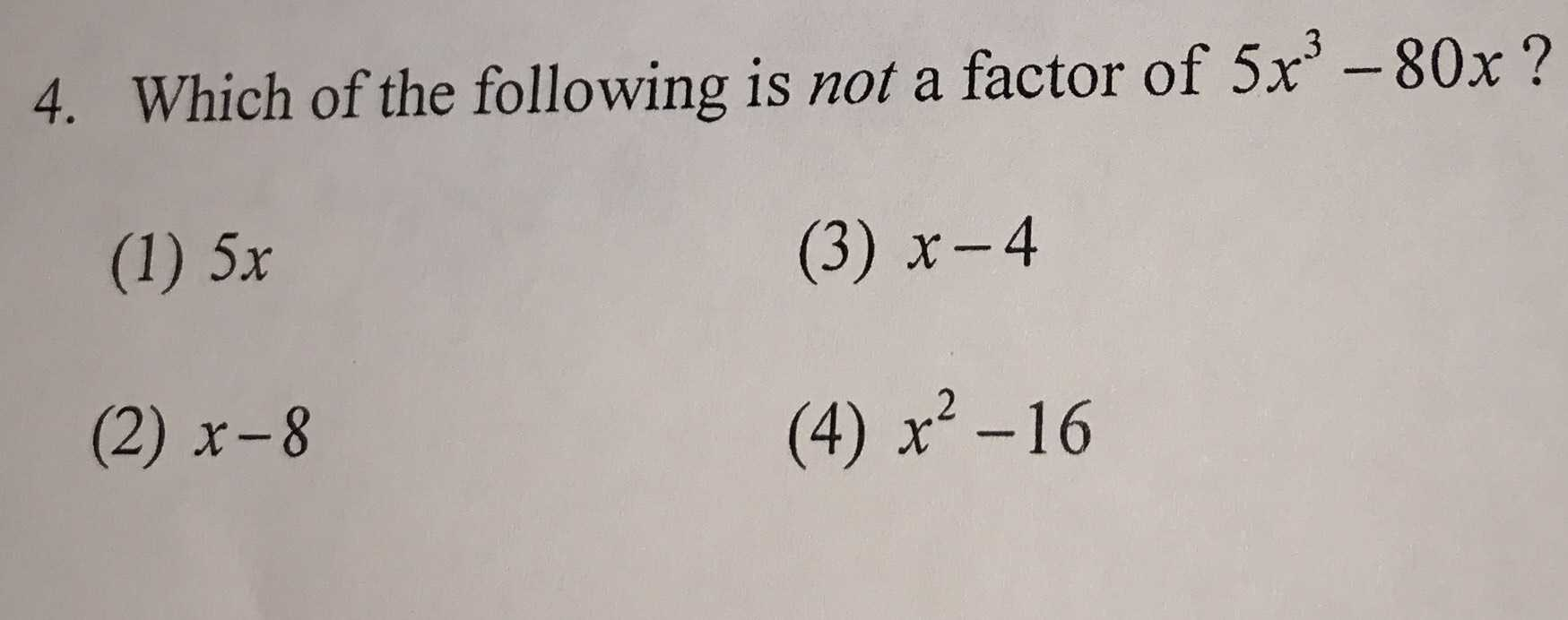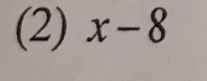### ¿Todavía tienes preguntas de matemáticas?

Pregunte a nuestros tutores expertos
Algebra
Pregunta4. Which of the following is not a factor of $$5 x ^ { 3 } - 80 x$$ ?

(1) $$5 x$$ (3) $$x - 4$$ (2) $$x - 8$$ (4) $$x ^ { 2 } - 16$$